In this report we describe a scheme for a quantum interface between superconducting qubits and optical photons and how to use such interfaces to coherently transfer quantum information optically between spatially remote superconducting qubits. Superconducting quantum devices have recently received a surge of interest and can now perform sophisticated manipulations on qubits and microwave photons locally on chip and are strong candidate for scalable quantum computing1,2,3. A coherent quantum transfer between distant superconducting qubits can thus serve as the underlying architecture for a genuine quantum internet. The key innovation of our scheme is to employ a magneto-mechanical interaction to provide strong coupling between the quantum state of a superconducting flux qubit (SQ) and a mechanical resonator (MR), which in turn couples via radiation pressure to an optical resonator (OR). By driving the SQ we are able to bring it into resonance with the magneto-mechanically coupled MR. By also optically driving the optomechanical system we can modulate the optomechanical coupling strength. Using realistic parameters we show that this configuration can achieve a high fidelity coherent swap between the quantum state of the SQ and the OR in an individual node.

In Fig. 1 a detailed design for such an opto-magneto-mechanical system is shown where the optical field in a Fabry Perot cavity is coupled via a Bulk Acoustic Wave mechanical resonance (BAW), which, via a magnetostrictive collar, is coupled magnetically to a SQ. By coupling two nodes via an optical fibre we create a small quantum network and we demonstrate a protocol to transfer the SQ quantum states between the nodes with high fidelity.

We begin with the quantum interface within a single node for coherent transfer of the superconducting and optically encoded quantum information and following that we analyse coherent quantum transfer between distant superconducting devices. The interface consists of an OR supporting one optical cavity mode and one mechanical vibrational mode and a SQ. The optical cavity mode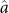has a resonance frequency ω and an intrinsic decay rate κi. The resonance frequency and relaxation rate of mechanical motion mode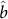are ωm and γm. To achieve the magneto-mechanical coupling between the SQ and MR we consider that the MR is partly made from a magnetostrictive material like Terfenol-D, which, in the presence of a magnetic field expands in our design, Fig. 1, the magnetostrictive material forms a collar around the BAW mechanical mode to allow magnetic field driving of the mechanical motion. This kind of magnetomechanical coupling has been used for magnetic field sensing and can be quite substantial4. Indeed this magneto-mechanical coupling is considerably stronger 40–400 MHz, than the proposed transfer rates using piezoelectric setups ~6 MHz5. Moreover, our design provides excellent mode overlap between the BAW mechanical vibrational mode and the expansion/contraction of the magnetostrictive collar. This should allow even larger magneto mechanical couplings than those previously reported. The SQ can be modeled as a two-level system (TLS) with the transition frequency ωq driven by a classical microwave field with frequency ωd. The frequency ωq can be tuned via a bias magnetic field. The optomechanical coupling can be described by the interaction Hamiltonian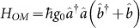, where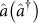and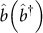are the photon and phonon annihilation (creation) operators for the optical and mechanical modes respectively and g0 is the optomechanical coupling rate. In the frame rotating at the frequency of driving field (on resonance with the SQ), the flux qubit with excited(ground) states |e〉(|g〉) can be modelled as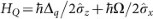, where Δq = ωq − ωd and the Pauli operators are defined as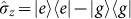and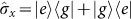. We assume that the flux qubit is driven by a classical field detuned by Δq from each SQ's transition frequency and with Rabi frequencies Ω. The macroscopic persistent current Ip circulating within each flux qubit induces a magnetic field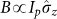dependent on the quantum state of each flux qubit6 (see the supplementary information). This magnetic field actuates the motion of the nearby mechanical resonator. This magneto-mechanical interaction is governed by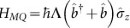, where Λ Ipxzp is the magnetomechanical-qubit coupling rate,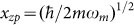is the zero-point width of the mechanical fluctuations with effective mass m. At this point we would like to note that while strong coupling between mechanical motion and a superconducting qubit has been achieved in17, this has not been achieved with a massive mechanical resonator that also allows coupling to light. The magnetic coupling we are proposing here allows for sufficiently strong qubit-mechanics coupling even for massive mechanical oscillators, such as the dilational mode of Bragg mirror we consider here.

When the flux qubit is put close to a magneto-optomechanical system (coupled MR + OR), they form a three-body quantum system whose total Hamiltonian is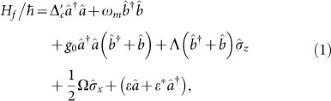where the external coherent field ε drives the optical cavity mode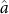and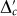is the detuning of this driving from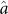. Setting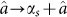and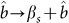, we then linearize the optomechanical interaction8,9 and then enter the dressed basis of the flux qubit by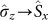,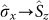10 (see supplementary information for the detail of the transformation). The reduced Hamiltonian for the node takes the form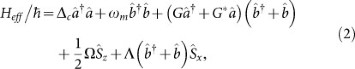with G = αsg0 and αss) are the steady-state values of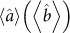, while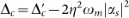, with the parameter η = g0m, αs ≈ ε*/(Δc + ), βs ≈ −η|αs|2 and κ is the optical cavity damping rate. Note that the parameters Δc, Ω and G are tunable while wm and Λ are not. The total detuning between the flux qubit and the driving is shifted due to the small βs and is given by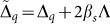. The small cubic term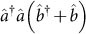is negligible because it only causes fast but small oscillation. Throughout our investigation below, we assume that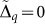, but is included in Hamiltonian HQ for generality.To avoid confusion, here we use different notations for the spin operators of the flux qubit in two different basis. Using this reduced Hamiltonian (2), we show below that we can reversibly swap a quantum state between the flux qubit and the optical cavity.

We now present our numerical results showing the coherent swapping of the quantum state between the flux qubit and the photon within a single node. In much of the literature in optomechanics the radiation pressure interaction is linearised as we have done in Eq. (2), where, via the large optical drive, strong optomechanical coupling can be achieved11. We have numerically verified that this linearisation is accurate for our three-body system, see the supplementary materials. The SQ is initially prepared in the excited state |ψqi = |e〉 and the OR in the photonic vacuum state |ψoi = |0〉 and the mechanical resonator is in a thermal state at temperature T ~ 10 mK, with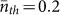, so that the unwanted decoherence induced by a large mechanical excitation is greatly suppressed. The optical field, mechanical mode and flux qubit interact resonantly and the resulting three-body Rabi oscillation is shown in Fig. 2. After a half Rabi oscillation, at κt1 = 0.39, the occupation of the SQ is transferred to the photon with a fidelity of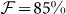(corresponding to na = 0.92) where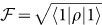. If we continue the evolution the quantum state swaps back to the SQ with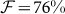at κt2 = 0.85. If we initially cool the mechanical resonator to nb = 0, the fidelity can increase to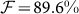at κt2 = 0.41 and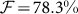at κt2 = 0.84. Our concrete design uses currently accessible parameters for each of the components and can achieve a high fidelity for microwave-to-optical conversion in a single node. Previous optomechanical12,13, or solid-state14, studies have proposed more abstract schemes for this interconversion, while Andrews et al.15, have demonstrated a classical MW-optical interconversion which operates at ~10% efficiency.

We now show how to to perform a coherent quantum transfer between the flux qubits in two distant nodes connected by an optical fibre. We consider the setup as shown in Fig. 3(a), where two identical interface nodes each with separate SQ, MR and OR elements and associated quantities labeled by j = 1, 2. ORj couples to a nearby optical fiber with a coupling rate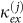and connects with the other node via this fiber in a cascaded fashion (via the optical circulators). Thus the total decay rate becomes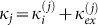and we assume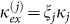with 0 ≤ ξj ≤ 1. The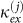denote the couplings into the waveguide connecting the two distant nodes. The master equation describing the cascaded two-node network capturing all forms of dissipation is16,17,18,19,20,21,22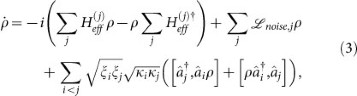where the Lindblad term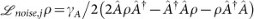with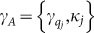and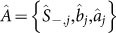describes the open system dynamics of the optomechanical resonators and flux qubits of the jth node. Because all interested processes finish within a time much smaller than the ring-down time of the mechanical resonator, we neglect the mechanical decoherence. The second line breaks time-reversal symmetry and models the cascaded quantum transfer from the node i to the node j (i < j), or reversely, where κj is the jth optical cavity decay rate and ξj represents photon loss in the transfer (we initially take ξj ≈ 1). We can also connect many nodes using the optical fiber as a quantum bus. Such a quantum network allows one to transfer a quantum state between two distant nodes. We show the simplest case consisting of two nodes only and assume that these two nodes are identical so that ξ1 = ξ2 = ξ. Currently, a superconducting flux qubit with a decay rate of γq/2π ~ kHz is available using existing technology23,24,25. This decay rate γq is much smaller than that of the optical cavity.

The distant transfer of quantum information is shown in Fig. 3(b). We assume a small decay rate for the flux qubit γq/2π = 0.02 MHz. When the effective Raman coupling ζ = ΛG/(Δc − ωm), is much smaller than the decay rate κ/2, we find that the constant coupling rates Λ and G allow for a transfer of quantum information from node 1 to node 2 with a high fidelity. This is an advantage over the complicated time-varying modulation of these rates required in related protocols16,17,18,19,20,21,22. Since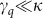, it is possible to transfer quantum information between SQs using weak coupling rates Λ and G. The occupation swapped to the photon from the flux qubit within the donator node quickly transfers to the photon in the acceptor node and then to the acceptor flux qubit. The high-frequency oscillations in the populations are due to the large detuning in the three-body Raman transition. As shown in Fig. 3(b), the state |e〉 is transferred with the fidelity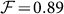corresponding to 80% occupation from the qubit 1 to the qubit 2 for constant coupling rates Λ = 4κ, G = 1.8 κ and Δc = 0.1ωm yielding |ζ| = 0.22 κ. The fidelity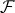to successfully transfer the quantum state is limited by the decay rate γq of the flux qubit and is approximately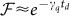, where td is the time to complete the maximal transfer. Therefore, a large κex but a small γq is preferable for a high fidelity transfer. We note that during the transfer the symmetric linear combination of both optical cavity fields is hardly excited while the antisymmetric one is only weakly excited. In Fig. 3(b) we consider the case that all the photonic excitation decaying from the optical cavity A is collected by the optical fiber and then is transferred to the flux qubit in the node B via the optical cavity B as shown in Fig. 3(b) (dashed). However, in reality there is a small probability for photon leakage either in the process of cavity-fibre coupling or scattering loss within the fibre itself. In this case, the fidelity of the transfer can be lower. We investigate how well the state can be transferred from qubit A to qubit B for ξ < 1, as shown in Fig. 3(b) (solid). For a loss corresponding to ξ = 0.9, we still can transfer the quantum state with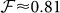corresponding to a probability of 65% from node A to node B. We would like to emphasize that our scheme is not a sequential state transfer of SQ to BAW to the cavity mode. Rather our scheme uses the mechanics to act as an intermediary between the SQ and cavity field and the detuned Raman drive allows an efficient qubit to optical state transfer without significant mechanical excitation at any one time.
All of the requirements to implement our magneto-mechanical superconducting qubit-optical quantum interface can be met with present day technology. These requirements are primarily: (i) it is desirable to have large mechanical resonance frequency ωm to reduce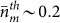and with pre-cooling to have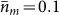initially. The state transfer fidelity is poor when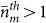; (ii) high transfer fidelity requires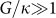, i.e. strong optomechanical coupling11,26 and (iii) we must achieve relatively strong magnetic coupling between the mechanics and flux qubit. Our design is depicted in Fig. 1, where one end mirror of the FP is constructed of a semiconductor multilayer Bragg circular mirror for light at λ = 1064 nm, made from AlGaAs27 (ρ = 4200 kg/m3, thickness t = 7 μm and radius r = 5 μm28. With a Finesse F ~ 2 × 105 and cavity length L = 10 μm, the effective mass of the BAW mode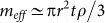, the fundamental BAW mode frequency (νm = vL/2t where vL is the longitudinal velocity of sound in the semiconductor, vL ~ 5130 m/s, zero-point motional extent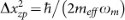, temperature T = 10 mK and bare optomechanical coupling rate (g0 = ωλΔxzp/L) are: meff ~ 770 pg, ωm/2π ~ 366 MHz,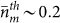, g0/2π ~ 8.3 kHz. To achieve large enough coupling rates G/κ ~ 1.8 with a cavity detuning Δc/κ = 0.1ωm, we must drive the FP cavity with an optical power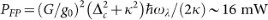, which is an acceptable power level for such an optical cavity. We note that with these parameters |αs| ~ 14, 000. The magneto-mechanical actuation is achieved by using a collar of magneto-strictive material (e.g. Terfenol-D), surrounding the moving mirror. When this collar sits in the local magnetic field of the flux qubit (|Blocal| ~ 6.7 nT), the collar exerts stress on the mirror, coupling to the BAWmode. Refs. 4, 29, use a similar arrangement that achieves an extremely large magneto-mechanical coupling strength Γ/2π ~ 1.2 GHz. In our design we assume a more modest coupling strength which is 2-orders of magnitude smaller Λ ~ 4κ = 2π × 40 MHz. This small coupling strength can greatly relax the requirement in the design of the structure. To avoid light interfering with the operation of the flux qubit one may arrange an aperture for the light to pass through contained within the flux qubit loop. Typically, the transition frequency of a flux qubit is much higher than that of a mechanical resonator. This large frequency difference prevents the quantum information exchange between them. To create an effective coupling, a coherent magnetic field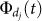is applied to strongly drive the flux qubit. As a result, the flux qubit oscillates at a Rabi frequency which matches the mechanical resonance frequency and this enhances their mutual coupling by several orders of magnitude4. This is physically achievable as a microwave pulse of P = −112 dBm propagating in an open transmission line is large enough to drive a flux qubit oscillating at about Ω/2π = 57 MHz for Ip = 195 nA30. Thus a pulse of P = −92 dBm (also used in30) can provide a Rabi frequency 570 MHz. Therefore, flux qubit Rabi frequencies of hundreds MHz is possible using existing technology. On the other hand, the available enhancement of the zero-point optomechanical coupling is limited by the usable photon number |αs|2 in the cavity. Note that the design shown in Fig. 1 is not restricted for operation at a particular optical wavelength and could be suitably modified to operate at the telecommunications wavelength of 1, 550 nm.